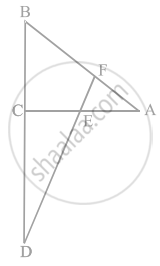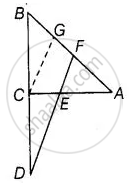# In figure, line segment DF intersect the side AC of a triangle ABC at the point E such that E is the mid-point of CA and ∠AEF = ∠AFE. Prove that BDCD=BFCE. - Mathematics

Sum

In figure, line segment DF intersect the side AC of a triangle ABC at the point E such that E is the mid-point of CA and ∠AEF = ∠AFE. Prove that (BD)/(CD) = (BF)/(CE).#### Solution

Given ΔABC, E is the mid-point of CA and ∠AEF = ∠AFE

To prove: (BD)/(CD) = (BF)/(CE)Construction: Take a point G on AB such that CG || EF

Proof: Since, E is the mid-point of CA.

∴ CE = GF   .....(i)

In ΔACG, CG || EF and E is mid-point of CA.

So, CE = GF  [By mid-point theorem]  .....(ii)

Now, In ΔBCG and ΔBDF, CG || EF

∴ (BC)/(CD) = (BG)/(GF) .......[By basic proportionality theorem]

⇒ (BC)/(CD) = (BF - GF)/(GF)

⇒ (BC)/(CD) = (BF)/(GF) - 1

⇒ (BC)/(CD) + 1 = (BF)/(CE)  .....[From equation (ii)]

⇒ (BC + CD)/(CD) = (BF)/(CE)

⇒ (BD)/(CD) = (BF)/(CE)

Hence proved.

Concept: Basic Proportionality Theorem (Thales Theorem)
Is there an error in this question or solution?

#### APPEARS IN

NCERT Mathematics Exemplar Class 10
Chapter 6 Triangles
Exercise 6.4 | Q 16 | Page 75

Share